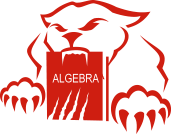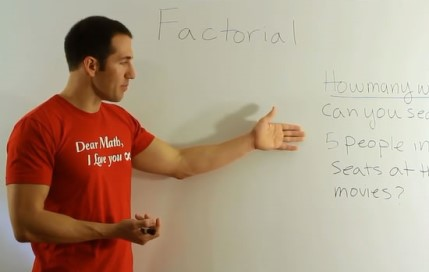##A strong Algebra background is a prerequisite for further study in mathematics and science, and for students studying a wide variety of college majors, College Algebra is part of their graduation requirements. Professors teaching College Algebra assume that their students have mastered Pre-Algebra, Elementary Algebra (Algebra 1), and Intermediate Algebra (Algebra 2).

The time constraints that exist in a traditional lecture based course, make it impossible for an instructor to slow down enough to explain in detail much of the prerequisite information that is required to follow the examples in a College Algebra course. As a result, many students find themselves lost in class. They simply need more examples, more time with those examples, and a more detailed set of explanations with those examples. That is where College Algebra videos can help.

There are hundreds of traditional Algebra textbooks in print today, but it is hard for many students to read a dry Algebra textbook. Quality Algebra tutoring would be the best solution for students who need additional help, but quality Algebra tutoring is often very expensive, hard to find, and inconvenient. A comprehensive set of College Algebra videos is an affordable alternative.

Panther Algebra strives to fill the need for free, quality Algebra help, that is easily accessible. All of the College Algebra videos featured on the site are recorded in high definition. The content is neatly organized into a simple to navigate chapter structure, and our responsive website can be accessed on any device anywhere an internet connection exists. Stop wasting valuable time searching for the help you need; start using Panther Algebra today.## Meet the Instructor

Dane McGuckian is a university mathematics instructor with more than 15 years of experience and the owner/creator of PantherAlgebra.com and STATSprofessor.com. Over the course of Dane's career, he has worked as a tutor, a high school teacher, a community college professor, and a university instructor. Teaching mathematics in these different settings has helped Dane learn to reach a diverse set of students by making complicated ideas and procedures seem simple.

## Chapters at a Glance

A prerequisite chapter and 7 information filled chapters and subchapters!

### 0.  Prerequisite Topics

This chapter covers, in detail, many of the prerequisite topics which must be mastered before starting College Algebra.

Please click the section you want to work on in oreder to access the videos.

### 1.  Equations & Inequalities

This chapter covers the techniques required to find solutions for various types of equations. The topics include: linear equations, quadratic equations, complex numbers, radical equations, higher order polynomial equations, and inequalities.

### 2.  Graphs in the Cartesian Plane

This chapter covers the techniques required to graph equations in the rectangular coordinate system. The topics include: distance, slope, midpoint, intercepts, symmetry, lines, and circles.

### 3.  Functions

This chapter covers the properties of functions, a library of functions, and graphs of functions. The topics include: relations, the definition of a function, domain, range, the difference quotient, graphing functions, average rate of change, maxima, and transformations.

### 4.  Linear & Quadratic Functions

This chapter further explores the properties of functions, their graphs, and their applications. The topics include: linear functions, demand, revenue, quadratic functions, vertices, axes of symmetry, intercepts, domain, range, extrema, and applications of functions.

### 5.  Polynomial & Rational Functions

This chapter covers polynomials and rational functions. The topics include: polynomial functions, the degree of a polynomial, graphing polynomials, zeros, multiplicity, turning points, end behavior of graphs,  domain, range, vertical asymptotes, horizontal asymptotes, slant (or oblique) asymptotes, graphing rational functions, rational and polynomial inequalities.

### 6.  Exponential & Log Functions

This chapter covers exponential and log functions. The topics include: function composition, domain of a composite function, inverse functions, one-to-one functions, graphs of inverse functions, finding inverse functions, graphing exponential functions, solving exponential equations, writing exponential equations into log form, graphing logarithmic functions, solving logarithmic equations, writing log equations into exponential form, properties of logs, and equations of quadratic form.

### 7.  Systems of Equations

This chapter covers some techniques to solve systems of equations. The topics include: solving systems of linear equations by substitution, solving systems of linear equations by elimination, and solving nonlinear systems by substitution and elimination.